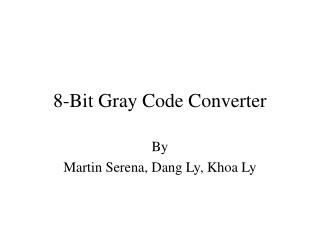DownloadDownload Presentation8-Bit Gray Code Converter

# 8-Bit Gray Code Converter

Download Presentation## 8-Bit Gray Code Converter

- - - - - - - - - - - - - - - - - - - - - - - - - - - E N D - - - - - - - - - - - - - - - - - - - - - - - - - - -
##### Presentation Transcript

1. 8-Bit Gray Code Converter By Martin Serena, Dang Ly, Khoa Ly

2. Overview • Gray Code Background • Delegated Duties • Method of Design • Target Specifications • Simulation Results • Block Diagram • Schematics, Symbols, Layouts, and Simulations • Design References • Conclusion

3. Gray Code Background • Conversion works in both directions • Binary  Gray , Gray  Binary • One bit changes from number to number • Not arithmetic • Not weighted (e.g. 222120) • Limits the amount of error that can occur when several bits change between numbers • No limit to number of converted bits

4. Binary to Gray Code Conversion 1 + 1 + 0 + 0 (BC) • MSB does not change as a result of conversion • Start with MSB of binary number and add it to neighboring binary bit to get the next Gray code bit • Repeat for subsequent Gray coded bits 1 0 1 0 (GC)

5. Gray to Binary Code Conversion 1 0 1 0 (GC) + + + • MSB does not change as a result of conversion • Start with MSB of binary number and add it to the second MSB of the Gray code to get the next binary bit • Repeat for subsequent binary coded bits 1 1 0 0 (BC)

6. Delegated Duties • Martin – Binary to Gray Conversion, Gray to Binary Conversion (XOR gates) • Dang – Binary/Gray Output Selection (MUXs) • Khoa – Binary Code Counter, Parallel-to-Parallel Shift Register (D flip-flops)

7. Method of Design • Decided on an initial load capacitance (Cin) • Partitioned the circuit into different propagation delay times according to gate/device requirements, and divided propagation delay times amongst the individual gates and devices • Created the symbol and layout for out each type of gate (XOR, MUX, NAND) • Connected gate symbols to create device symbols • Connected gate layouts to create device layouts • Connected device symbols to create circuit schematics, and connected device layouts to create circuit layouts

8. Target Specifications • Conversion: • Binary Code to Gray Code • Gray Code to Binary Code • Propagation delay times: • XOR (each): 0.4 nS • MUX (each): 0.3 nS • D flip-flop (each): 0.63 nS (worst-case fall time) • Technology specs (size): • Minimum Channel Width = 1.5 m • Minimum Channel Length = 0.6 m • Power < ¼ Watt • Clock Speed = 200 MHz • Total area as small as possible

9. Simulation Results • Successfully converts binary and Gray codes • Propagation Delay • XOR (each): 0.338 nS (worst-case) • MUX (each): 0.35 nS (worst-case) • D flip-flop (each): 1.14 nS (worst-case fall time) • Technology specs (size) • Transistor Lengths: 0.6 m • XOR: Wp = 3.9 m Wn = 3.75 m • MUX: Wp = 6 m Wn = 3 m • D Flip-Flop: Wp = 18 m Wn = 10 m

10. Simulation Results • Power (using the power meter) • 39.94 mW • Clock Speed • 200 MHz • Total Area • Gray code converter: 6.03E-4 cm2 • Counter: 10.2E-4 cm2

11. Block Diagram

12. XOR Schematic

13. XOR Symbol

14. XOR Layout

15. XOR Extracted

16. XOR LVS Report

17. XOR Test Bench

18. XOR Transient Analysis

19. XOR Threshold

20. MUX Schematic

21. MUX Symbol

22. MUX Layout

23. MUX Extracted

24. MUX LVS Report

25. MUX Test Bench

26. MUX Transient Analysis

27. MUX Transient Analysis

28. NAND3 Schematic

29. NAND3 Symbol

30. NAND3 Layout

31. NAND3 Extracted

32. NAND3 LVS Report

33. NAND3 Test Bench

34. NAND3 Transient Analysis

35. D Flip-Flop Schematic

36. D Flip-Flop Symbol

37. D Flip-Flop Layout

38. D Flip-Flop Extracted

39. D Flip-Flop LVS Report

40. D Flip-Flop Test Bench

41. D Flip-Flop Transient Analysis

42. Counter Schematic

43. Counter Symbol

44. Counter Layout

45. Counter Extracted

46. Counter LVS Report

47. Counter Transient Analysis

48. Gray Code Converter Schematic

49. Gray Code Converter Symbol

50. Gray Code Converter Layout